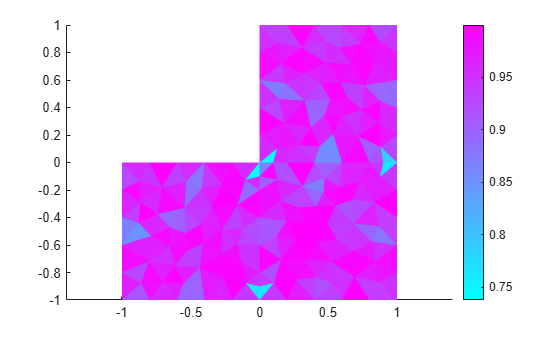# jigglemesh

(Not recommended) Jiggle internal points of triangular mesh

This page describes the legacy workflow. New features might not be compatible with the legacy workflow. For the corresponding step in the recommended workflow, see `generateMesh`.

## Syntax

``p1 = jigglemesh(p,e,t)``
``p1 = jigglemesh(p,e,t,Name,Value)``

## Description

example

````p1 = jigglemesh(p,e,t)` jiggles the triangular mesh by adjusting the node point positions. Typically, the quality of the mesh increases after jiggling.```

example

````p1 = jigglemesh(p,e,t,Name,Value)` jiggles the mesh using one or more `Name,Value` arguments.```

## Examples

collapse all

Create a triangular mesh of the square geometry by using `initmesh`. To avoid jiggling, call `initimesh` with the `Jiggle` value set to `off`.

`[p,e,t] = initmesh('lshapeg','Jiggle','off'); `

Evaluate quality of the mesh elements using the `pdetriq` function.

`q = pdetriq(p,t); `

Plot the mesh.

`pdeplot(p,e,t,'XYData',q,'ColorBar','on','XYStyle','flat')`Jiggle the mesh using the default parameter values. Plot the result.

```p1 = jigglemesh(p,e,t); q = pdetriq(p1,t); pdeplot(p1,e,t,'XYData',q,'ColorBar','on','XYStyle','flat')```Now jiggle the original mesh again, this time using 50000 iterations.

```p2 = jigglemesh(p,e,t,'Opt','off','Iter',5e4); q = pdetriq(p2,t); pdeplot(p2,e,t,'XYData',q,'ColorBar','on','XYStyle','flat')```## Input Arguments

collapse all

Mesh points, specified as a 2-by-`Np` matrix. `Np` is the number of points (nodes) in the mesh. Column `k` of `p` consists of the x-coordinate of point `k` in `p(1,k)` and the y-coordinate of point `k` in `p(2,k)`. For details, see Mesh Data as [p,e,t] Triples.

Mesh edges, specified as a 7-by-`Ne` matrix, where `Ne` is the number of edges in the mesh. An edge is a pair of points in `p` containing a boundary between subdomains, or containing an outer boundary. For details, see Mesh Data as [p,e,t] Triples.

Mesh elements, specified as a 4-by-`Nt` matrix. `Nt` is the number of triangles in the mesh.

The `t(i,k)`, with `i` ranging from 1 through `end - 1`, contain indices to the corner points of element `k`. For details, see Mesh Data as [p,e,t] Triples. The last row, `t(end,k)`, contains the subdomain number of the element.

### Name-Value Arguments

Specify optional pairs of arguments as `Name1=Value1,...,NameN=ValueN`, where `Name` is the argument name and `Value` is the corresponding value. Name-value arguments must appear after other arguments, but the order of the pairs does not matter.

Before R2021a, use commas to separate each name and value, and enclose `Name` in quotes.

Example: `p1 = jigglemesh(p,e,t,'Iter',Inf)`

Optimization method, specified as the comma-separated pair consisting of `'Opt'` and `'mean'`, `'minimum'`, or `'off'`.

Jiggling a mesh moves each mesh point not located on an edge segment towards the center of mass of the polygon formed by the adjacent triangles. The optimization method controls how many times `jigglemesh` repeats this process:

• If `Opt` is `'off'`, `jigglemesh` repeats this process `Iter` times. The default value of `Iter` in this case is 1.

• If `Opt` is `'mean'`, `jigglemesh` repeats this process until the mean triangle quality stops increasing significantly or until the maximum number of iterations is reached. The default value of `Iter` in this case is 20.

• If `Opt` is `'minimum'`, `jigglemesh` repeats this process until the minimum triangle quality stops increasing significantly or until the maximum number of iterations is reached. The default value of `Iter` in this case is 20.

Example: ```p1 = jigglemesh(p,e,t,'Opt','off','Iter',1000); ```

Data Types: `char` | `string`

Maximum number of iterations, specified as the comma-separated pair consisting of `'Iter'` and a positive number. The default value depends on the `Opt` argument value. If `Opt` is set to `'mean'` (default) or `'minimum'`, the default maximum number of iterations is 20. If `Opt` is set to `'off'`, the default maximum number of iterations is 1.

Example: ```p1 = jigglemesh(p,e,t,'Opt','off','Iter',1000);```

Data Types: `double`

## Output Arguments

collapse all

Modified mesh points, returned as a 2-by-`Np` matrix. `Np` is the number of points (nodes) in the mesh. Column `k` of `p` consists of the x-coordinate of point `k` in `p(1,k)` and the y-coordinate of point `k` in `p(2,k)`. For details, see Mesh Data as [p,e,t] Triples.

## Version History

Introduced before R2006a